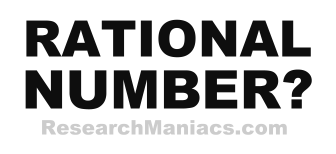Is 43 a Rational Number?The number 43 is a rational number if 43 can be expressed as a ratio, as in RATIOnal.

A quotient is the result you get when you divide one number by another number. For 43 to be a rational number, the quotient of two integers must equal 43.

In other words, for 43 to be a rational number, 43 must be able to be expressed as a ratio where both the numerator and the denominator are integers (whole numbers).

The number 43 can be expressed as 43/1 where 43 is the numerator and 1 is the denominator.

Thus, the answer to the question "Is 43 a rational number?" is YES.

Rational Number?
Enter another number to see if it is rational:

Is 44 a Rational Number?
See information about the next number on our list.